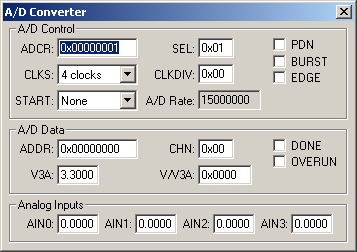Products Download Events Support Videos All Product Families ARM7, ARM9, and Cortex-M3 Products C16x, XC16x, and ST10 Products C251 and 80C251 Products Cx51 and 8051 Products Modified Anytime In the Last 7 Days In the Last 14 Days In the Last 30 Days In the Last 90 Days In the Last 6 Months

# Peripheral Simulation

For NXP (founded by Philips) LPC2194 — A/D Converter (4-Channels)

Simulation support for this peripheral or feature is comprised of:

• Dialog boxes which display and allow you to change peripheral configuration.
• VTREGs (Virtual Target Registers) which support I/O with the peripheral.

These simulation capabilities are described below.

### A/D Converter DialogThe A/D Converter dialog displays the status registers for all of the A/D converters supported by this device. The Analog Input Channels, listed at the bottom of the dialog, allow you to specify the voltage for each analog input.

A/D Control

• ADCR (A/D Control Register) contains the following control bits for the A/D controller:
• CLKS (Conversion Clocks)selects the number of clocks used for each conversion in Burst mode and the bit accuracy.
• START controls when and if the an A/D conversion is started.
• SEL selects which pins of the analog input (AINn) are to be sampled and converted.
• CLKDIV (Clock Divisor) is the value (minus 1) divided into the VPB clock to produce the A/D Rate.
• A/D Rate is the A/D clock rate.
• PDN (Power Down) is set id the A/D is operational. If reset, the A/D is in power down mode.
• (BURST) is set to use the number of clocks specified in BURST. If reset, conversions are software controlled and requires 11 clocks.
• (EDGE) is set to start the conversion on a rising edge. If reset, conversion starts on a falling edge.

A/D Data

• ADDR (A/D Data Register) contains the following bits for controllong A/D data conversion:
• CHN (Channel) is the channel containing the converted bits.
• V3A ()
• V/V3A ()
• DONE is set when the A/D conversion completes.
• OVERRUN is set if one or more conversions were overwritten before the current conversion completed.

• (AINn) (Analog Input Channels n) are used for setting voltage input to the ADC. These inputs are alternate functions for Port pins. The text box displays the analog voltage value for each input channel.

### AINx VTREGData Type: float

```AIN0 = 0.000  /* Analog Input 0 = 0.000 volts */
```

You may create a debugger signal function to periodically change the value of the A/D input. The following signal function increases the A/D Channel 1 input voltage by 0.1 volts each second.

```signal void AIN1_sig (void) {
float f;

for (f = 0.0; f < 5.0; f += 0.1)
{
swatch (1.0);                  // Delay 1 second
AIN1 = f;                      // Set AIN1 Voltage
}
}
```

### V3A VTREGData Type: float